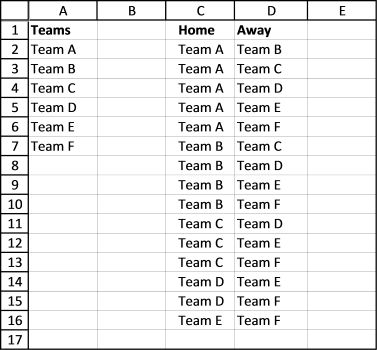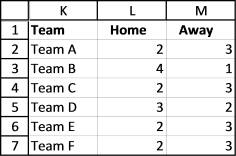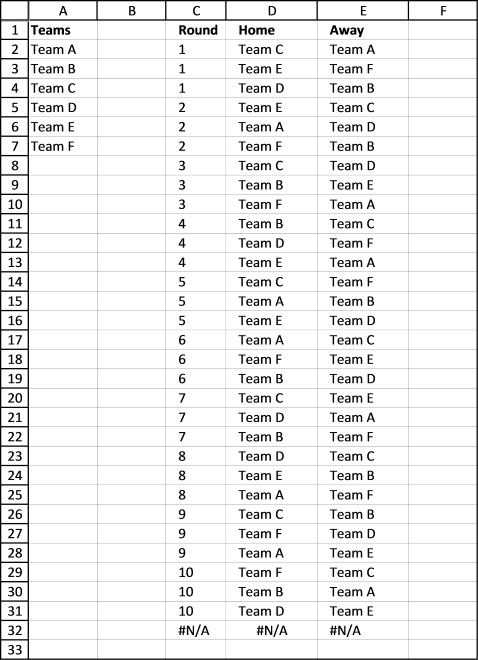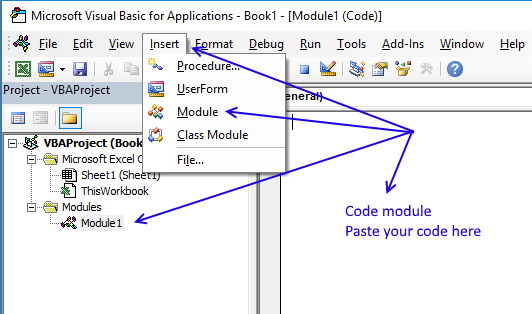Author: Oscar Cronquist Article last updated on June 16, 2021According to Wikipedia, a round-robin tournament is a competition where all plays all. Excel is a great platform for building a round-robin tournament table and keeping scores.

You can use these custom functions below for creating a table for tennis, soccer, chess, bridge, or whatever sport/competition schedule you want. At the very end of this post are instructions on how to use the custom functions. Let's start.

1. Basic scheduling - each team plays once against another team

The following VBA code creates a schedule where each team plays once against another team.

'Name
Function roundrobin(rng As Range)
'Get Digital Help https://www.get-digital-help.com/round-robin-tournament/

'Dimension variables and declare data types
Dim tmp() As Variant, k As Long
Dim i As Long, j As Long

'ReDimension array variable tmp
ReDim tmp(1 To (rng.Cells.Count / 2) * (rng.Cells.Count - 1), 1 To 2)

'Save 1 to variable k
k = 1

'Schedule everyone with everyone
'For ... Next statement
'Go from 1 to the number of cells in range variable rng
For i = 1 To rng.Cells.Count

'Go from i + 1 to the number of cells in range variable rng
For j = i + 1 To rng.Cells.Count

'Save value stored in worksheet based on variable i to array variable tmp based on variable k
tmp(k, 1) = rng.Cells(i)

'Save value stored in worksheet based on variable j to array variable tmp based on variable k
tmp(k, 2) = rng.Cells(j)

'Add 1 to the number stored in variable k and save to variable k
k = k + 1

'Continue with next number stored in variable j
Next j

'Continue with next number stored in variable i
Next i

'Return array to worksheet
roundrobin = tmp

End FunctionAs you can see it is not very complicated and the first team has home matches all the time. But if home and away doesn't matter this could useful.

Another bad thing with this custom function is that it doesn't split the schedule into rounds. A team can't play twice in the same round, obviously.

Also if you want the schedule to be somewhat random, this custom function is not for you.

The next custom function takes care of these three issues.

2. Round-robin tournament - distributes home and away rounds evenly

This custom function creates a round-robin tournament. It tries to distribute home and away rounds evenly and teams are randomly placed in the schedule.

'Name User Defined Function and specify parameters
Function RoundRobin2(rng As Range)
'This custom function adds a team automatically if the number of teams is uneven.
'Get Digital Help https://www.get-digital-help.com/round-robin-tournament/

'Dimension variables and declare data types
Dim tmp() As Variant, k As Long, l As Integer
Dim i As Long, j As Long, a As Long, r As Long
Dim rngA As Variant, Stemp As Variant, Val As Long
Dim res, rngB() As Variant, result As String

'Transfer cell values to an array
rngA = rng.Value

'Check if the number of teams are even
If rng.Cells.Count Mod 2 = 0 Then

'Save cell values in variable rng to variable rngB
rngB = rng.Value

'Save 0 (zero) to l
l = 0

'Continue here if the number of teams are not even
Else

'Redimension array variable rngB
ReDim rngB(1 To UBound(rngA) + 1, 1 To 1)

'For... Next statement
For i = 1 To UBound(rngA)

'Save value in array variable rngA to array variable rngB
rngB(i, 1) = rngA(i, 1)
Next i

'Save character - to the last container in array variable rngB
rngB(UBound(rngB, 1), 1) = "-"

'Save 1 to variable l
l = 1
End If

'Redimension array variable tmp
ReDim tmp(1 To ((rng.Cells.Count + l) / 2) * (rng.Cells.Count + l - 1), 1 To 3)

'Randomize array
rngB = RandomizeArray1(rngB)

Val = (UBound(rngB, 1) / 2)

'Build schedule
For i = 2 To UBound(rngB, 1)

'Save 1 to variable a
a = 1

'For ... Next statement
'Go from 1 to half of the number of rows in array variable rng2
For r = 1 To (UBound(rngB, 1) / 2)

tmp(r + Val * (i - 2), 1) = i - 1
If (i - 1) Mod 2 = 1 Then
tmp(r + Val * (i - 2), 2) = rngB(a, 1)
tmp(r + Val * (i - 2), 3) = rngB(UBound(rngB, 1) - a + 1, 1)
Else
tmp(r + Val * (i - 2), 2) = rngB(UBound(rngB, 1) - a + 1, 1)
tmp(r + Val * (i - 2), 3) = rngB(a, 1)
End If
a = a + 1
Next r

'switch places for all values except the first one
For j = 2 To UBound(rngB, 1) - 1
Stemp = rngB(j, 1)
rngB(j, 1) = rngB(j + 1, 1)
rngB(j + 1, 1) = Stemp
Next j
Next i

'Return values in array variable tmp to worksheet
RoundRobin2 = tmp

End Function

This User Defined Function creates a random schedule split into rounds, home and away are also somewhat evenly distributed through the schedule.This table shows you how many times 6 teams play home and away for the entire tournament.3. Double round-robin tournament - User Defined Function

To make sure every team has as many home as away rounds competitors play each other twice. It is a "double" round-robin tournament. The way it works is all play all twice, once home and once away.

'Name User Defined Function and specify parameters
Function doubleroundrobin(rng As Range)
'Get Digital Help https://www.get-digital-help.com/round-robin-tournament/

'Dimension variables and declare data types
Dim tmp() As Variant, k As Long, l As Integer
Dim i As Long, j As Long, a As Long, r As Long
Dim rngA As Variant, Stemp As Variant, Val As Long
Dim res, rngB() As Variant, result As String, cc As Long

'Transfer values to an array
rngA = rng.Value

'Check if the number of teams are even
If rng.Cells.Count Mod 2 = 0 Then
rngB = rng.Value
l = 0
Else
ReDim rngB(1 To UBound(rngA) + 1, 1 To 1)
For i = 1 To UBound(rngA)
rngB(i, 1) = rngA(i, 1)
Next i
rngB(UBound(rngB, 1), 1) = "-"
l = 1
End If

cc = ((rng.Cells.Count + l) / 2) * (rng.Cells.Count + l - 1)

'Redimension array variable tmp
ReDim tmp(1 To cc * 2, 1 To 3)

'Randomize array
rngB = RandomizeArray1(rngB)

'Save half of the number of rows in array variable rngB to variable Val
Val = (UBound(rngB, 1) / 2)

'Build schedule
For i = 2 To UBound(rngB, 1)

a = 1
For r = 1 To (UBound(rngB, 1) / 2)
tmp(r + Val * (i - 2), 1) = i - 1
If (i - 1) Mod 2 = 1 Then
tmp(r + Val * (i - 2), 2) = rngB(a, 1)
tmp(r + Val * (i - 2), 3) = rngB(UBound(rngB, 1) - a + 1, 1)
Else
tmp(r + Val * (i - 2), 2) = rngB(UBound(rngB, 1) - a + 1, 1)
tmp(r + Val * (i - 2), 3) = rngB(a, 1)
End If
a = a + 1
Next r
For j = 2 To UBound(rngB, 1) - 1
Stemp = rngB(j, 1)
rngB(j, 1) = rngB(j + 1, 1)
rngB(j + 1, 1) = Stemp
Next j
Next i

'Copy schedule and change home to away and vice versa, this makes it a double round-robin tournament
For i = cc + 1 To cc * 2
tmp(i, 1) = UBound(rngB, 1) - 1 + tmp(i - cc, 1)
tmp(i, 2) = tmp(i - cc, 3)
tmp(i, 3) = tmp(i - cc, 2)
Next i

'Return values in array variable tmp to worksheet
doubleroundrobin = tmp

End FunctionHere is a table that shows you teams play as many home as away games.

4. Macro

The macros in the workbook below allow you to create a match schedule. Go to sheet Macro and follow instructions.Add teams or players to column A, then press with left mouse button on the "Round-robin tournament" button or "Double round-robin tournament".

A match schedule is created on a new sheet.Conditional formatting separates rounds with a line, it makes the table easier to read.

Round-robin tournament

Sub rr()
'Get Digital Help, https://www.get-digital-help.com/round-robin-tournament/

Dim Lrow As Long
Dim rng As Range, tmp() As Variant
Dim ws As Worksheet
Application.ScreenUpdating = False
Lrow = Worksheets("Macro").Range("A" & Rows.Count).End(xlUp).Row
Set rng = Worksheets("Macro").Range("A2:A" & Lrow)

tmp = RoundRobin2(rng)

'Insert new sheet

ws.Range("A1") = "Round"
ws.Range("B1") = "Home"
ws.Range("C1") = "Away"
ws.Range("A2").Resize(UBound(tmp, 1), 3) = tmp

ws.Range("A1").Resize(UBound(tmp, 1) + 1, 3).InsertIndent 1
Columns("A:C").EntireColumn.AutoFit
ws.Range("A1:C1000").Select
Selection.FormatConditions(Selection.FormatConditions.Count).SetFirstPriority
With Selection.FormatConditions(1).Borders(xlBottom)
.LineStyle = xlContinuous
.Weight = xlThin
End With
Selection.FormatConditions(1).StopIfTrue = False
Application.ScreenUpdating = True
End Sub

Double round-robin tournament

Sub droundrobin()
Dim Lrow As Long
Dim rng As Range, tmp() As Variant
Dim ws As Worksheet
Application.ScreenUpdating = False
Lrow = Worksheets("Macro").Range("A" & Rows.Count).End(xlUp).Row
Set rng = Worksheets("Macro").Range("A2:A" & Lrow)

tmp = doubleroundrobin(rng)

'Insert new sheet

ws.Range("A1") = "Round"
ws.Range("B1") = "Home"
ws.Range("C1") = "Away"
ws.Range("A2").Resize(UBound(tmp, 1), 3) = tmp

ws.Range("A1").Resize(UBound(tmp, 1) + 1, 3).InsertIndent 1
Columns("A:C").EntireColumn.AutoFit
ws.Range("A1:C1000").Select
Selection.FormatConditions(Selection.FormatConditions.Count).SetFirstPriority
With Selection.FormatConditions(1).Borders(xlBottom)
.LineStyle = xlContinuous
.Weight = xlThin
End With
Selection.FormatConditions(1).StopIfTrue = False
Application.ScreenUpdating = True
End Sub

This function moves values in an array randomly.

'Name User Defined Function
Function RandomizeArray1(Arr As Variant)

'Dimension variables and declare data types
Dim temp
Dim i As Long, j As Long, k As Long
Dim result As Variant

'For ... Next statement
For k = LBound(Arr, 1) To UBound(Arr, 1)

result = result & Arr(k, 1) & " "

Next k

'Add new line to variable result
result = result & vbNewLine

'For ... Next statement
For i = LBound(Arr, 1) To UBound(Arr, 1)

'Generate random value
j = Application.WorksheetFunction.RandBetween(LBound(Arr, 1), UBound(Arr, 1))

'Save value in array variable Arr to temp
temp = Arr(j, 1)
Arr(j, 1) = Arr(i, 1)
Arr(i, 1) = temp
For k = LBound(Arr, 1) To UBound(Arr, 1)
result = result & Arr(k, 1) & " "
Next k
result = ""
Next i

RandomizeArray1 = Arr

End Function

Get the Excel fileround-robin-tournamentv2.xlsm

5. How to use a custom function

If you want the vba code in your own workbook, do this.

1. Press Alt-F11 to open visual basic editor
2. Press with left mouse button on Module on the Insert menu
3. Copy and paste all custom functions above to the code module4. Exit visual basic editor (Alt+Q)
5. Save your workbook as a *.xlsm file

Now you can use the custom functions. Type your teams in a column. Select a blank cell range, 3 columns wide and many rows, you can extend this later if not all rounds show up.

Type =doubleroundrobin(cell_ref_to_your_teams), press and hold CTRL + Shift. Press Enter. Release all keys.